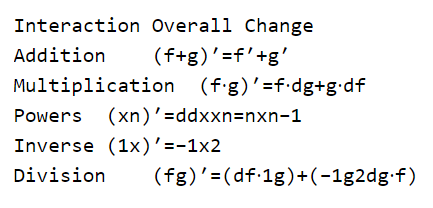# PyTorch — A General Introduction

Source: Deep Learning on Medium# PyTorch — A General Introduction

Pytorch is an open source deeplearning framework developed by Facebook. It is very interactive like Python and it is getting very popular in deeplearnig community. Easy to learn, easy to implement.

It has strong GPU support as result models run faster. Basics of Pytorch are tensors which are very similar to numpy. Tensors multiplication becomes very fast in GPU (Graphics Processing Units).

Pytorch has already many algorithms implemented. It becomes very easy to use these algorithms on any dataset.

# Matrix Multiplication

Matrix multiplication is very important in neural network. We can say that the basics of neural network is matrix multiplication since at every step we need to multiply tensors which are also a type of matrix

# Pytorch Comparison with Numpy

`import torchimport numpy as npprint("Tensor :","\n")torch.tensor([[2,3,5],[1,2,9]])Tensor :tensor([[2, 3, 5], [1, 2, 9]])print ("Numpy array :", "\n")np.array([[2,3,5],[1,2,9]])Numpy array :array([[2, 3, 5], [1, 2, 9]])`
• Tensor and Numpy array looks similar
• Lets generate some random array
`torch.rand(2,2)tensor([[0.7703, 0.4446], [0.0589, 0.2118]])np.random.rand(2,2)array([[0.56424161, 0.08179224], [0.57521072, 0.74610146]])`